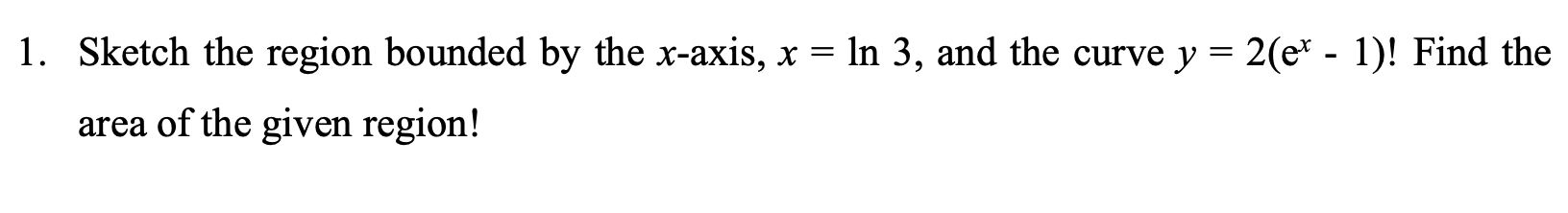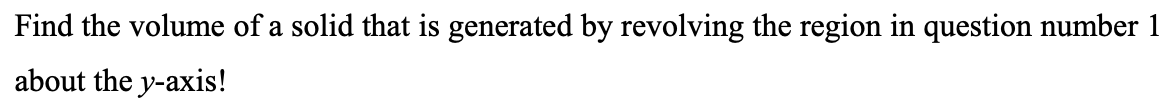### Create an Account

Already have account?

### Forgot Your Password ?

Home / Questions / 1. Sketch the region bounded by the x-axis, x = ln 3, and the curve y = 2(et - 1)! Find th...

# 1. Sketch the region bounded by the x-axis, x = ln 3, and the curve y = 2(et - 1)! Find the area of the given region! Find the volume of a solid that is generated by revolving the region in question n

1. Sketch the region bounded by the x-axis, x = ln 3, and the curve y = 2(et - 1)! Find the area of the given region!
Find the volume of a solid that is generated by revolving the region in question number 1 about the y-axis!Apr 14 2021 View more View Less

#### Answer (Solved)Subscribe To Get Solution